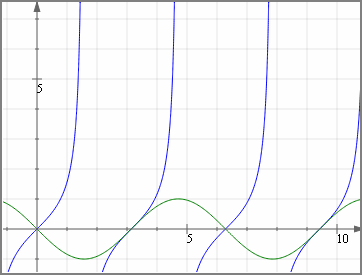Search IntMath
Close

# Online graph plotter using JSXGraph

By Murray Bourne, 03 Jun 2011

JSXGraph allows you to plot mathematical functions on any browser, and on any operating system.

You can now plot your own graphs using this online graphing calculator:

Plot your own graphs using JSXGraph

You can input many different functions, including:

• Straight lines and parabolas
• Trigonometric functions and their inverses
• Exponential and log functions, including base 10
• Hyperbolic functions

This JSXGraph plotter has similar functionality to my SVG-based plotter, but works in more browsers and systems.

JSXGraph works on iPhone and Android, too!

Here's a screen shot:Try it out and let me know if you have suggestions for improvement. The link again:

Plot your own graphs using JSXGraph

### Comment Preview

HTML: You can use simple tags like <b>, <a href="...">, etc.

To enter math, you can can either:

1. Use simple calculator-like input in the following format (surround your math in backticks, or qq on tablet or phone):
a^2 = sqrt(b^2 + c^2)
(See more on ASCIIMath syntax); or
2. Use simple LaTeX in the following format. Surround your math with $$ and $$.
$$\int g dx = \sqrt{\frac{a}{b}}$$
(This is standard simple LaTeX.)

NOTE: You can mix both types of math entry in your comment.